Select Page

# CBSE Maths 12 Science MCQ Application of Integrals Solutions in English

CBSE Maths 12 Science MCQ Application of Integrals Solutions in English to enable students to get Solutions in a narrative video format for the specific question.

Expert Teacher provides CBSE Maths 12 Science MCQ Application of Integrals Solutions through Video Solutions in English language. This video solution will be useful for students to understand how to write an answer in exam in order to score more marks. This teacher uses a narrative style for a question from Application of Integrals not only to explain the proper method of answering question, but deriving right answer too.

Please find the question below and view the Solution in a narrative video format.

Question:

Solution Video in English:

## Similar Questions from CBSE, 12th Science, Maths, Application of Integrals

Question 1 : Using the method of integration find the area of the region bounded by lines:
2x + y = 4, 3x - 2y = 6 and x - 3y + 5 = 0.

Question 2 : Find the area enclosed by the parabola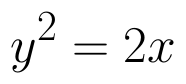and the line x - y = 4. (View Answer Video)

Question 3 : Find the area of smaller region bounded by the ellipse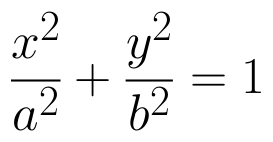and the line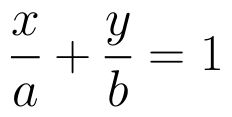. (View Answer Video)

Question 4 : Find the area of the smaller region bounded by the ellipse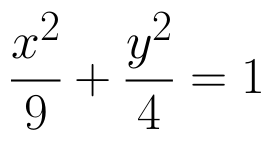and the line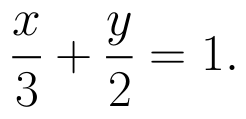(View Answer Video)

Question 5 : Using integration find the area of region bounded by the triangle whose vertices are (-1, 0), (1, 3) and (3, 2). (View Answer Video)

### Continuity and Differentiability

Question 1 : Differentiate the function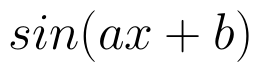with respect to x. (View Answer Video)

Question 2 : Differentiate the function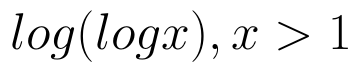with respect to x. (View Answer Video)

Question 3 : Differentiate w.r.t.x the function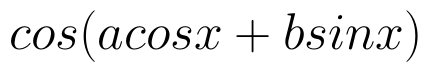, for some constant a and b. (View Answer Video)

Question 4 : Using the fact that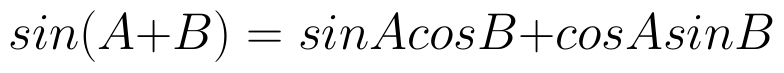and the differentiation, obtain the sum formula for cosines. (View Answer Video)

Question 5 : Differentiate the function w.r.t.x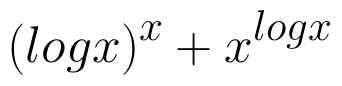. (View Answer Video)

### Application of Derivatives

Question 1 : Equation of normal to the curve x+y=x^y where it cuts x-axis; is (View Answer Video)

Question 2 : The line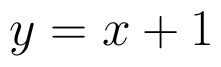is a tangent to the curve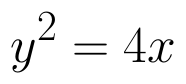at the point. (View Answer Video)

Question 3 : It is given that  at x=1, the function attains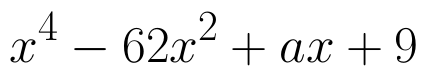its maximum value on the interval[0,2]. Find the value of a? (View Answer Video)

Question 4 : A balloon, which always remains spherical on inflation, is being inflated by pumping in 900 cubic centimetres of gas per second. Find the rate at which the radius of the balloon increases when the radius is 15 cm. (View Answer Video)

Question 5 : The normal  to the curve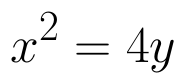passing (1,2) is____________. (View Answer Video)

### Differential Equations

Question 1 : Solve the differential equation: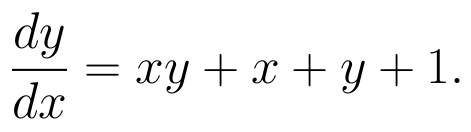(View Answer Video)

Question 2 : Solve the differential equation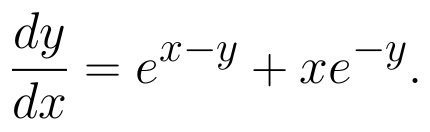(View Answer Video)

Question 3 : Solve the following differential equation :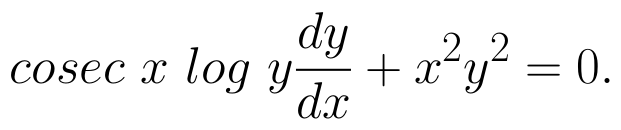(View Answer Video)

Question 4 : Find the sum of the order and the degree of the following differential equation: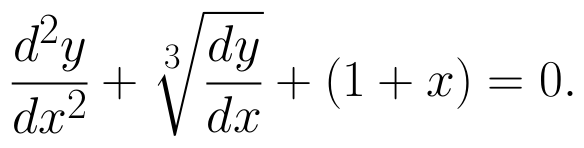(View Answer Video)

Question 5 : Write the differential equation representing the curve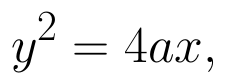where a is an arbitrary constant. (View Answer Video)